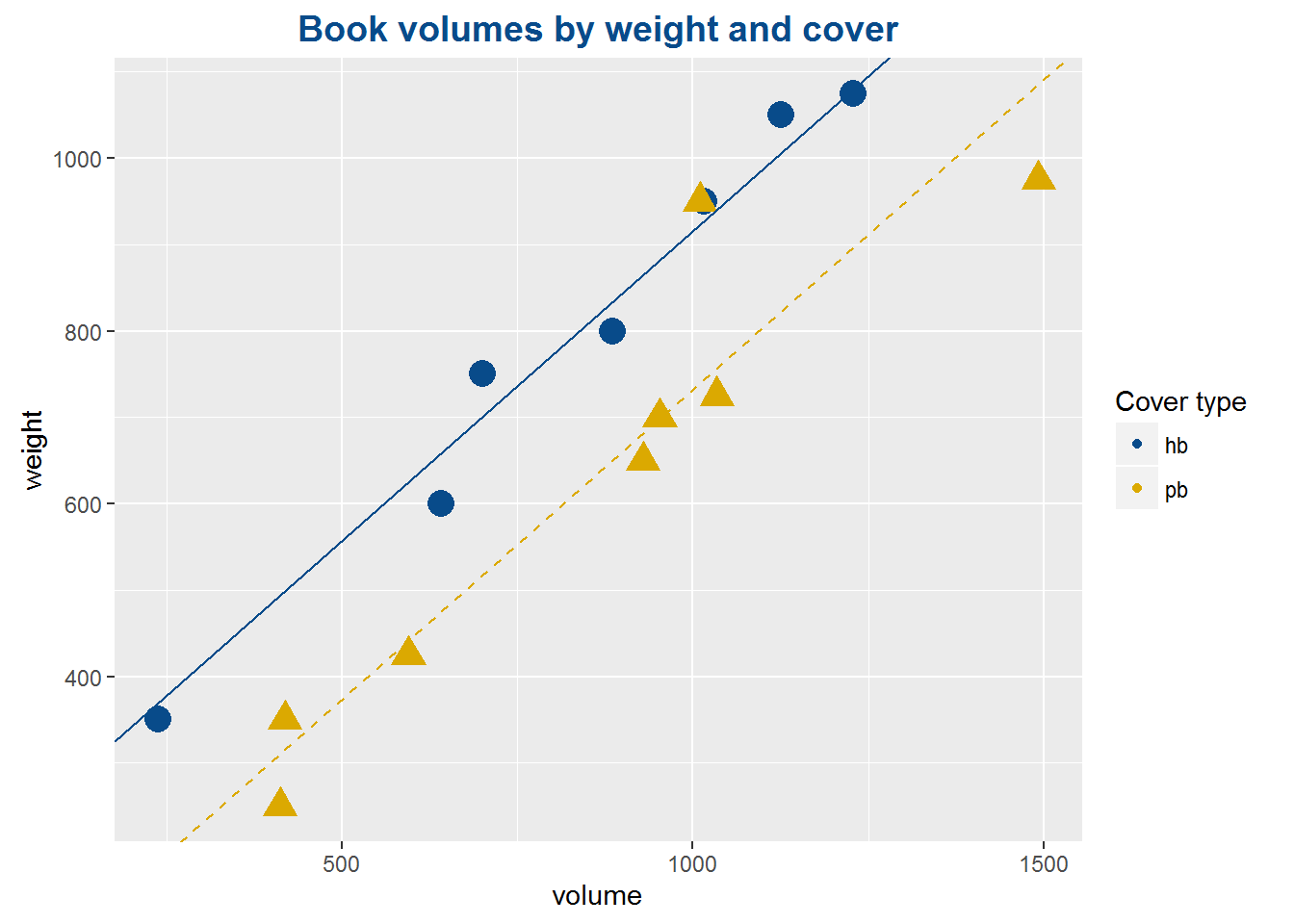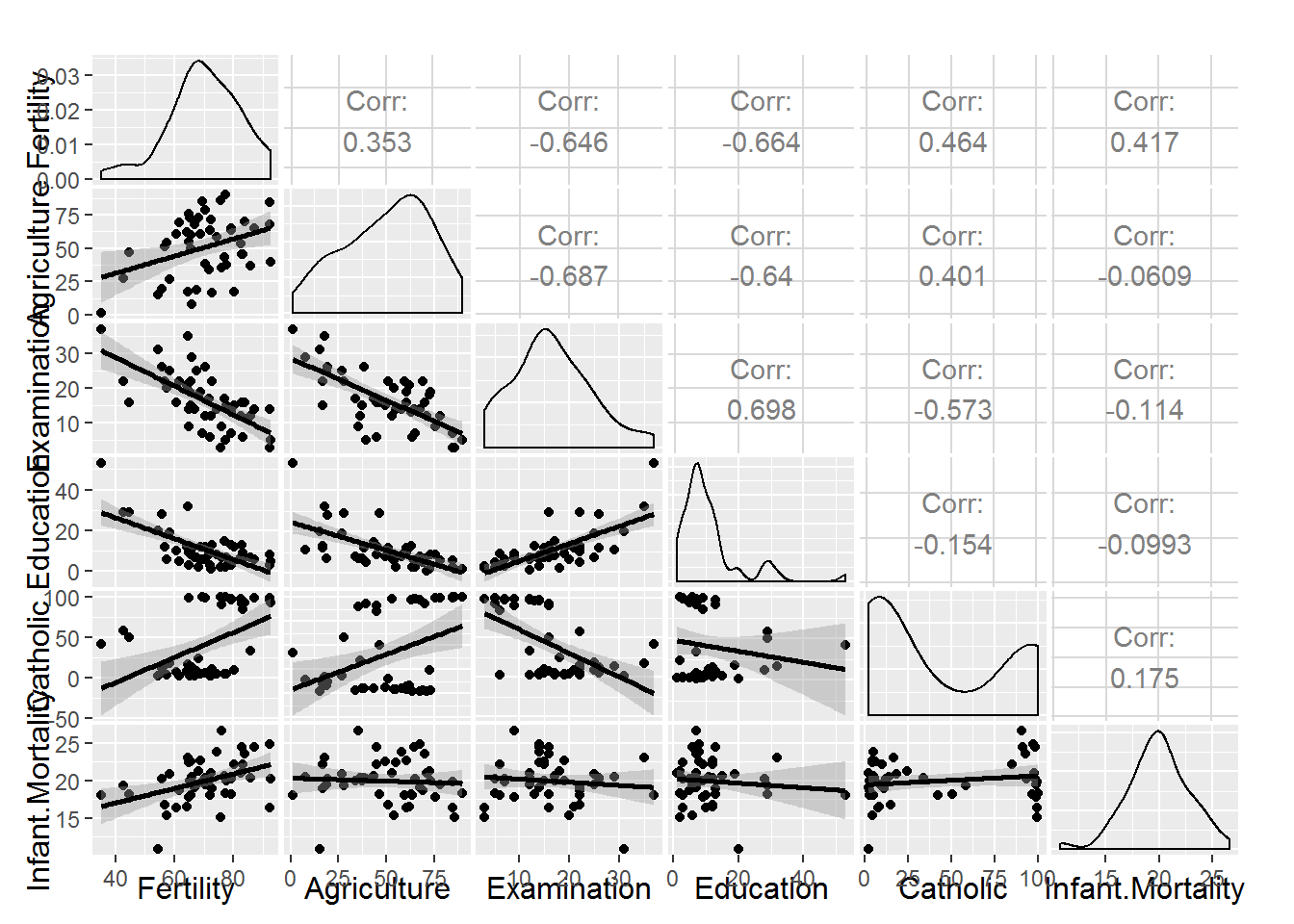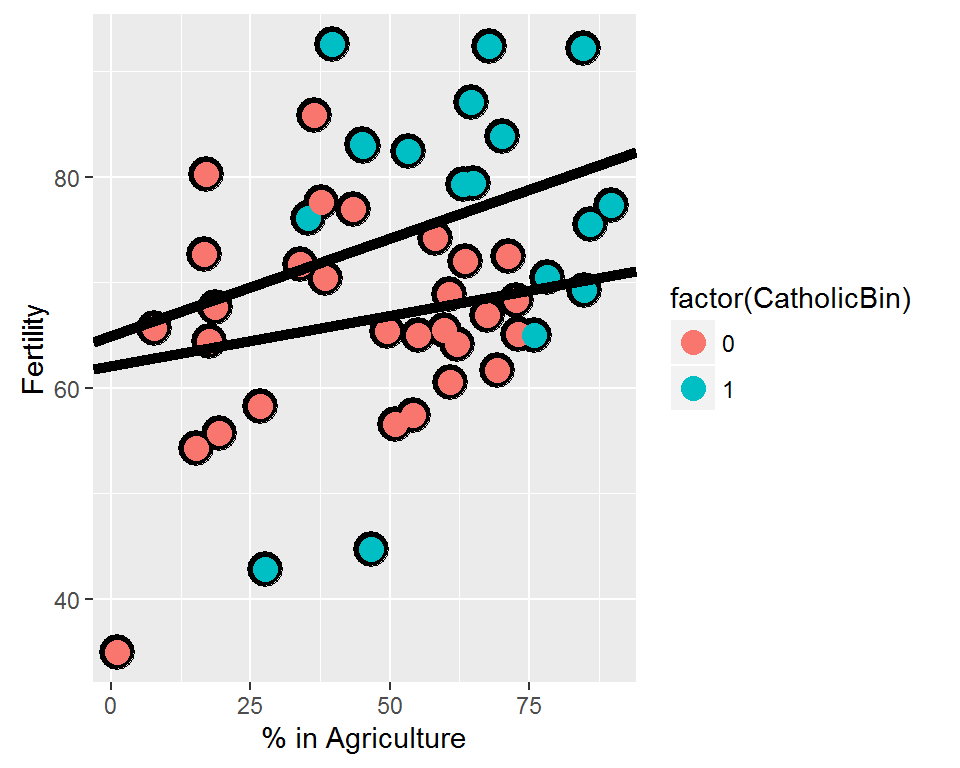# Multiple Linear Regression

If you see an error in the article, please comment or drop me an email.

# Conditions for multiple linear regression

• linear relationship between each (numerical) explanatory variable and the response – checked using scatterplots of y vs. each x, and residuals plots of residuals vs. each x

• nearly normal residuals with mean 0 – checked using a normal probability plot and histogram of residuals

• constant variability of residuals – checked using residuals plots of residuals vs. fitted, and residuals vs. each x

• independence of residuals (and hence observations) – checked using a scatterplot of residuals vs. order of data collection (will reveal non-independence if data have time series structure)

• Add a boxplot of residuals vs each explanatory variable to identify changes in variability

Multiple linear regression is a method allowing to explain a response variable by several other variables.

# Gaussian elimination

The general technique is to pick one predictor and to replace all other variables by the residuals of their regressions against that one.

The mean of a variable is equal to its regression against the constant, 1

``## Warning: package 'lmtest' was built under R version 3.3.2``
``````data("galton")
lm(child ~ 1,data=galton)``````
``````##
## Call:
## lm(formula = child ~ 1, data = galton)
##
## Coefficients:
## (Intercept)
##       68.09``````
``mean(galton\$child)``
``##  68.08847``

Regression in many variables amounts to a series of regressions in one.

### Model with only an intercept

`lm(child ~ 1, data=galton)`

### Model with only a slope

`lm(child ~ parent - 1, data=galton)`

# MLR with a factor variable – The Books example

For instance, let us have a look at a dataset containing measurements of books: weight, cover type, volume, etc. If we try to explain `weight` by `cover` and `volume`, we are doing multiple linear regression.

Note that `cover` is a factor variable (with values `0` and `1`), which makes this example quite particular. As a factor variable, `cover` will influence only the height of the regression line, but not its slope.

``````data("allbacks") #from DAAG package

#Fit the model
book_mlr <- lm(weight~volume + cover, data=allbacks)
book_mlr_summary <- summary(book_mlr)

#Show the plot
ldbcol <- c("#084B8A","#DBA901")
g <- ggplot(data=allbacks,aes(x=volume,y=weight,
pch=cover, colour=cover, size=6)) +
geom_point() +
guides(size=FALSE,pch=FALSE,colour=guide_legend("Cover type")) +
theme(plot.title = element_text(family = "sans", color="#084B8A",
face="bold", size=14, hjust=0.5)) +
scale_colour_manual(values=ldbcol) +
labs(title="Book volumes by weight and cover") +
geom_abline(slope=book_mlr_summary\$coefficients,
intercept=book_mlr_summary\$coefficients+book_mlr_summary\$coefficients*0,
colour=ldbcol, linetype=1) +
geom_abline(slope=book_mlr_summary\$coefficients,
intercept=book_mlr_summary\$coefficients+book_mlr_summary\$coefficients*1,
colour=ldbcol, linetype=2)
g``````The summary of the fit is similar to the one with only one predictor. As you can see below, hb (=hardback) is the reference for the `cover` factor variable. (if you insert a zero for cover, you find the results only for hardcover books).

``print(book_mlr_summary)``
``````##
## Call:
## lm(formula = weight ~ volume + cover, data = allbacks)
##
## Residuals:
##     Min      1Q  Median      3Q     Max
## -110.10  -32.32  -16.10   28.93  210.95
##
## Coefficients:
##               Estimate Std. Error t value Pr(>|t|)
## (Intercept)  197.96284   59.19274   3.344 0.005841 **
## volume         0.71795    0.06153  11.669  6.6e-08 ***
## coverpb     -184.04727   40.49420  -4.545 0.000672 ***
## ---
## Signif. codes:  0 '***' 0.001 '**' 0.01 '*' 0.05 '.' 0.1 ' ' 1
##
## Residual standard error: 78.2 on 12 degrees of freedom
## Multiple R-squared:  0.9275, Adjusted R-squared:  0.9154
## F-statistic: 76.73 on 2 and 12 DF,  p-value: 1.455e-07``````

Interpretation:

• All else held constant, for each 1cm3 increase in volume, the model predicts the books to be heavier on average by 0.72 grams

• All else held constant, the model predicts that paperback books weigh 184.05 grams lower than hardcover books, on average.

• Intercept hardcover books (cover=0) with no volume (volume=0) are expected to weigh 198 grams on average (meaningless in this context)

Conditions:

• This model assumes that paperback and hardcover books have the same slope for the relationship between their volume and weight. If this assumption is not reasonable, we would include an interaction variable.

# How to calculate the adjusted correlation coefficient – The States Example

``````states <- read.csv("http://bit.ly/dasi_states")
pov_slr <- lm(poverty ~ female_house, data = states)
summary(pov_slr) #summary of the model``````
``````##
## Call:
## lm(formula = poverty ~ female_house, data = states)
##
## Residuals:
##     Min      1Q  Median      3Q     Max
## -5.7537 -1.8252 -0.0375  1.5565  6.3285
##
## Coefficients:
##              Estimate Std. Error t value Pr(>|t|)
## (Intercept)    3.3094     1.8970   1.745   0.0873 .
## female_house   0.6911     0.1599   4.322 7.53e-05 ***
## ---
## Signif. codes:  0 '***' 0.001 '**' 0.01 '*' 0.05 '.' 0.1 ' ' 1
##
## Residual standard error: 2.664 on 49 degrees of freedom
## Multiple R-squared:  0.276,  Adjusted R-squared:  0.2613
## F-statistic: 18.68 on 1 and 49 DF,  p-value: 7.534e-05``````
``anova(pov_slr) #anova analysis of the model``
``````## Analysis of Variance Table
##
## Response: poverty
##              Df Sum Sq Mean Sq F value    Pr(>F)
## female_house  1 132.57 132.568  18.683 7.534e-05 ***
## Residuals    49 347.68   7.095
## ---
## Signif. codes:  0 '***' 0.001 '**' 0.01 '*' 0.05 '.' 0.1 ' ' 1``````

R2 will increase with each explanatory variable added to the model, regardless of whether or not the added variable is a meaningful predictor of the response variable. Therefore we use adjusted R2, which applies a penalty for the number of predictors included in the model, to better assess the strength of a multiple linear regression model:

`R_adj^2 <- 1 - ((SSE/SST) * ((n-1)/(n-k-1)))`

• SSE = Sum of unexplained squares

• SST = Total sum of squares

• n = sample size

• k = number of predictors

``````pov_mlr <- lm(poverty ~ female_house + white, data = states)
pov_mlr_anova <- anova(pov_mlr)
SSE <- pov_mlr_anova\$`Sum Sq` #SSE = UNexplained variability!!
SST <- sum(pov_mlr_anova\$`Sum Sq`)
n <- nrow(states)
k <- 2
R_adj_2 <- 1 - ((SSE/SST) * ((n-1)/(n-k-1)))
``##  0.2636785``
``summary(pov_mlr)\$adj.r.squared``
``##  0.2636785``

## Difference between R_squared and adjusted R_squared

R_squared tells us the percentage of variability in the response variable explained by the model.

Adjusted R_squared has the same starting point, but also applies a penalty for the number of predictors.

# Exploratory analysis in multiple regression – The Swiss Example

### Explore the variables with a pairs plot

For our example, we will use the Swiss dataset, measuring fertility and socio-economic indicators for each of 47 French-speaking provinces of Switzerland in 1888.

``````data(swiss)
g <- ggpairs(swiss, lower = list(continuous = "smooth"))
g````````#Source: https://github.com/DataScienceSpecialization/courses``

### Check the impact of each potential explanatory variable

``summary(lm(Fertility ~ . , data = swiss))\$coefficients #Use period "." to input all remaining variables``
``````##                    Estimate  Std. Error   t value     Pr(>|t|)
## (Intercept)      66.9151817 10.70603759  6.250229 1.906051e-07
## Agriculture      -0.1721140  0.07030392 -2.448142 1.872715e-02
## Examination      -0.2580082  0.25387820 -1.016268 3.154617e-01
## Education        -0.8709401  0.18302860 -4.758492 2.430605e-05
## Catholic          0.1041153  0.03525785  2.952969 5.190079e-03
## Infant.Mortality  1.0770481  0.38171965  2.821568 7.335715e-03``````

Let us focus on the Agriculture variable. If we disregard all other variables, what happens to the impact of the Agriculture variable?

``summary(lm(Fertility ~ Agriculture , data = swiss))\$coefficients``
``````##               Estimate Std. Error   t value     Pr(>|t|)
## (Intercept) 60.3043752 4.25125562 14.185074 3.216304e-18
## Agriculture  0.1942017 0.07671176  2.531577 1.491720e-02``````

The coefficient for agriculture changed signs and is now positive. This result underlines the importance of eliminating cofounders. Multiple linear regression is a dynamic process.

The pairs plot clearly showed which variables are correlated. Among them:

• examination and education, at .6984

• agriculture and education, at -.6395

### What happens if a completely unnecessary variable is added to the model?

``````z <- swiss\$Agriculture+swiss\$Education
#no added value in z as it is just a combination of pre-existing variables
lm(Fertility ~ . + z , data = swiss)``````
``````##
## Call:
## lm(formula = Fertility ~ . + z, data = swiss)
##
## Coefficients:
##      (Intercept)       Agriculture       Examination         Education
##          66.9152           -0.1721           -0.2580           -0.8709
##         Catholic  Infant.Mortality                 z
##           0.1041            1.0770                NA``````

The new unnecessary variable z is marked NA.

### How to change the reference level in MLR with a factor variable?

``````data(InsectSprays);
summary(lm(count~spray,data=InsectSprays))``````
``````##
## Call:
## lm(formula = count ~ spray, data = InsectSprays)
##
## Residuals:
##    Min     1Q Median     3Q    Max
## -8.333 -1.958 -0.500  1.667  9.333
##
## Coefficients:
##             Estimate Std. Error t value Pr(>|t|)
## (Intercept)  14.5000     1.1322  12.807  < 2e-16 ***
## sprayB        0.8333     1.6011   0.520    0.604
## sprayC      -12.4167     1.6011  -7.755 7.27e-11 ***
## sprayD       -9.5833     1.6011  -5.985 9.82e-08 ***
## sprayE      -11.0000     1.6011  -6.870 2.75e-09 ***
## sprayF        2.1667     1.6011   1.353    0.181
## ---
## Signif. codes:  0 '***' 0.001 '**' 0.01 '*' 0.05 '.' 0.1 ' ' 1
##
## Residual standard error: 3.922 on 66 degrees of freedom
## Multiple R-squared:  0.7244, Adjusted R-squared:  0.7036
## F-statistic:  34.7 on 5 and 66 DF,  p-value: < 2.2e-16``````
``````#change reference level
spray2 <- relevel(InsectSprays\$spray, "C")
summary(lm(count~spray2,data=InsectSprays))``````
``````##
## Call:
## lm(formula = count ~ spray2, data = InsectSprays)
##
## Residuals:
##    Min     1Q Median     3Q    Max
## -8.333 -1.958 -0.500  1.667  9.333
##
## Coefficients:
##             Estimate Std. Error t value Pr(>|t|)
## (Intercept)    2.083      1.132   1.840   0.0702 .
## spray2A       12.417      1.601   7.755 7.27e-11 ***
## spray2B       13.250      1.601   8.276 8.51e-12 ***
## spray2D        2.833      1.601   1.770   0.0814 .
## spray2E        1.417      1.601   0.885   0.3795
## spray2F       14.583      1.601   9.108 2.79e-13 ***
## ---
## Signif. codes:  0 '***' 0.001 '**' 0.01 '*' 0.05 '.' 0.1 ' ' 1
##
## Residual standard error: 3.922 on 66 degrees of freedom
## Multiple R-squared:  0.7244, Adjusted R-squared:  0.7036
## F-statistic:  34.7 on 5 and 66 DF,  p-value: < 2.2e-16``````

# Including an interaction term

The introduction of an interaction term offers the possibility of fitting two regression lines with a specific slope and intercept.

When `x_2` is a dummy variable:

`beta_0 + (beta_1 * x_1) + (beta_2 * x_2)`

• if `x_2 = 0`: `beta_0 + (beta_1 * x_1)`

• if `x_2 = 1`: `(beta_0 + beta_2) + (beta_1 * x_1)`

This means that for both values of the dummy variable, the slope (`beta_1`) is the same, but the intercept is different.

When adding an interaction variable, the slopes and the intercept will be different:

`beta_0 + (beta_1 * x_1) + (beta_2 * x_2) + (beta_3 * x_1 * x_2)`

• if `x_2 = 0`: `beta_0 + (beta_1 * x_1)`

• if `x_2 = 1`: `(beta_0 + beta_2) + ( (beta_1 + beta_3) * x_1 )`

To add an interaction variable in R:

`lm(res_var ~ exp_var1 * exp_var2)`

Or see example below, including a response variable (Fertility) and two explanatory variables (Agriculture and CatholicBin). Note that CatholicBin is a dummy variable.### Determine whether including an interaction term makes sense

1. Fit a model without the interaction term
• `fit_wo <- lm(y ~ x + y:x, data=dataset)`
1. Fit a model with the interaction term
• `fit_w <- lm(y ~ x, data=dataset)`
1. Conduct a likelihood ratio test on both models
• `lrtest(fit_wo,fit_w)` (note that `lrtest()*` comes with the `lmtest` package)

In our Swiss data example:

``````#Fit model above without interaction term
fit_wo <- lm(Fertility ~ Agriculture + factor(CatholicBin), data = swiss)
lrtest(fit,fit_wo)``````
``````## Likelihood ratio test
##
## Model 1: Fertility ~ Agriculture * factor(CatholicBin)
## Model 2: Fertility ~ Agriculture + factor(CatholicBin)
##   #Df  LogLik Df  Chisq Pr(>Chisq)
## 1   5 -179.34
## 2   4 -179.48 -1 0.2792     0.5972``````